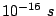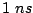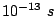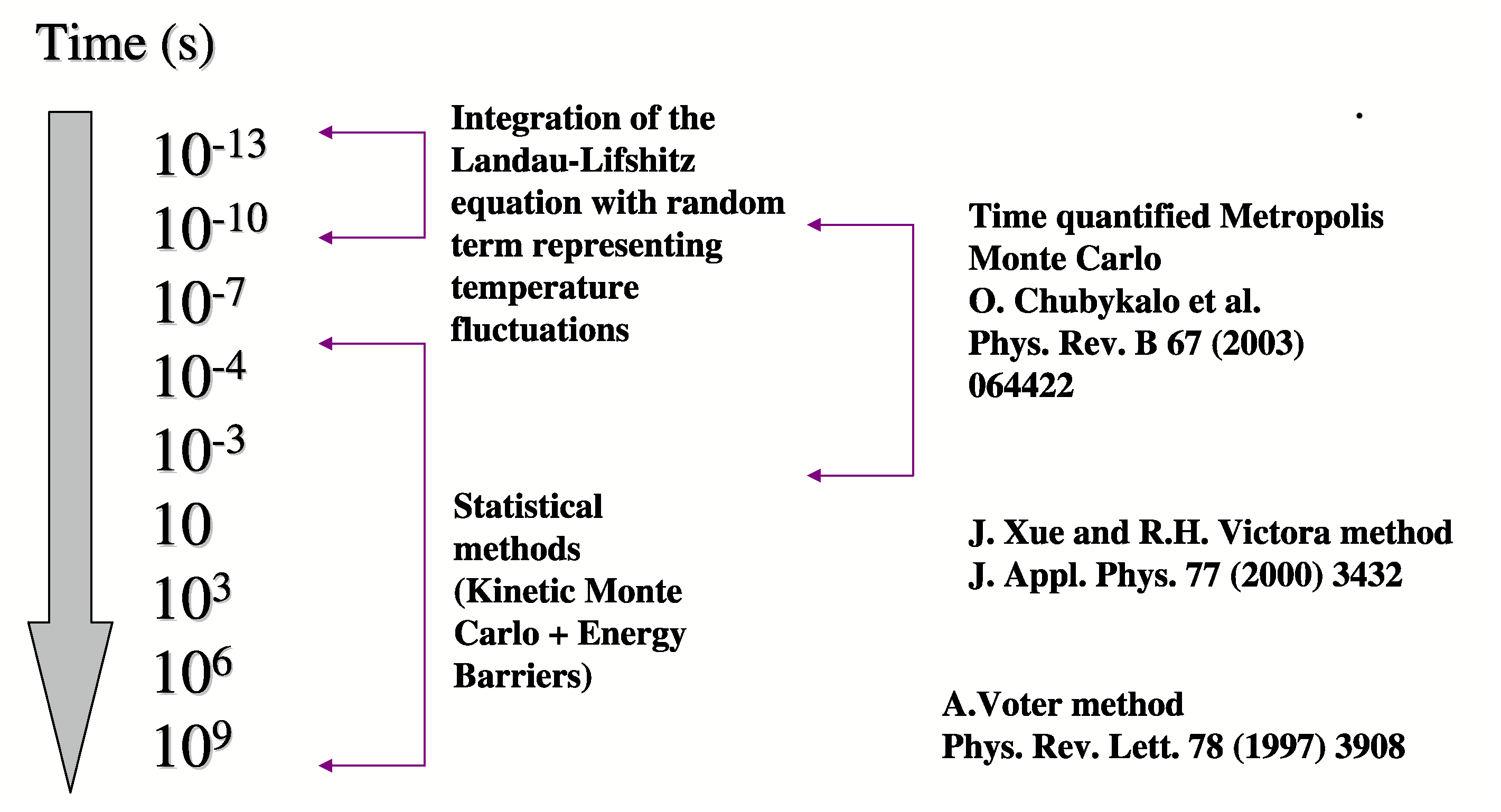From the point of view of simulation, the main parameter is the time-step of the numerical integration of the basic equation of motion (the Landau-Lifshitz-Gilbert equation). In the atomistic modeling, the time step is defined by the exchange interaction and is of the order of. Therefore, integrating the equation of motion at atomistic level, one can afford the description of small system up to the time of. In the micromagnetic systems the time-step has to be at least two orders of magnitude less than the frequency of precession of the magnetization. The resulting time-step is aboutand the reachable time simulations of tens of nanoseconds, far from interval of interest in most of the experimental situations. These limits depend on the system size and in the computer technology advances.